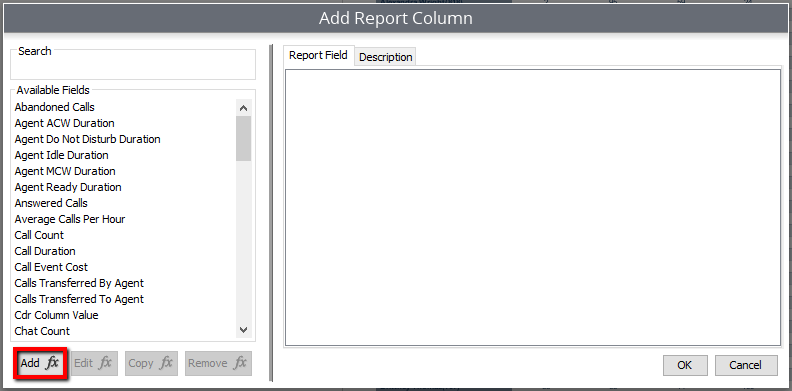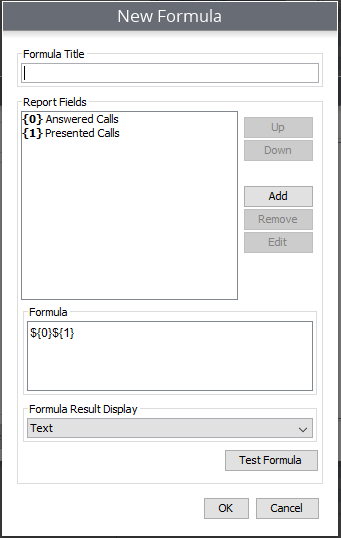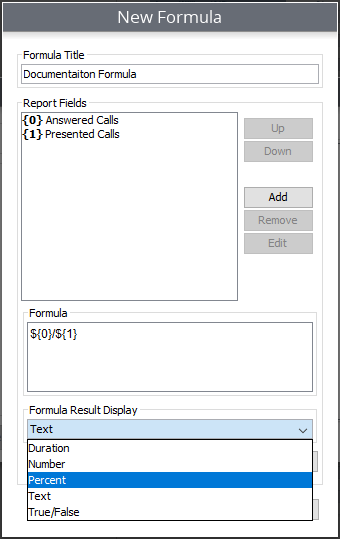# Creating Custom Formulas

This provides instructions on how to create custom formulas for a custom report. Access to this feature requires the Custom Reports module.

When creating and editing a report you are provided with complete flexibility. This means that you can either use the provided values as is, or you can adjust them to fit your needs.

However, if you are not able to find the value that fits your needs, or if you want to provide further depth to your values, you will also have the option to create your own formulas. This is done by selecting values combined with simple mathematical equations.

1. While creating or editing a report select "Add" to add a new column to the report
2. Select the "Add Formula" button## Selecting the Values

The "New Formula" window will appear and you can now begin to build your formula.

1. Start by naming your formula.
2. Now you can select the values that you will use to build your formula. Start by hitting "Add" on the right-hand side. You will now be presented with a window displaying the value options available for you to choose from
3. Select the desired value, then select "OK."

Repeat steps 2 and 3 until you have selected the values necessary to build your formula.

## Building the Formula

Once you have selected the desired values, you can now build your formula.

In the Formula window you will see the values that you have selected represented by a dollar sign and curly brackets surrounding a number (\${0}). The dollar sign signifies the beginning of a value and the number coincides with the value selected in the Report Fields section above.To create the formula, we will now have to create the mathematical equation. In this scenario we want to see a percentage of calls answered compared to calls presented. In the formula window, you would see \${0}\${1}. To achieve the desired result, a division symbol will be placed between the two values.

It would look like this \${0}/\${1}.

Once the formula is complete, select the Formula Result Display drop down, and select the desired result display.(i.e., duration, number, percent, etc.)You can also select Test Formula. This will assist in verifying that your equation makes sense.

Once your formula is complete, it will now be featured in your list of value options, meaning it can be used on future reports as well.